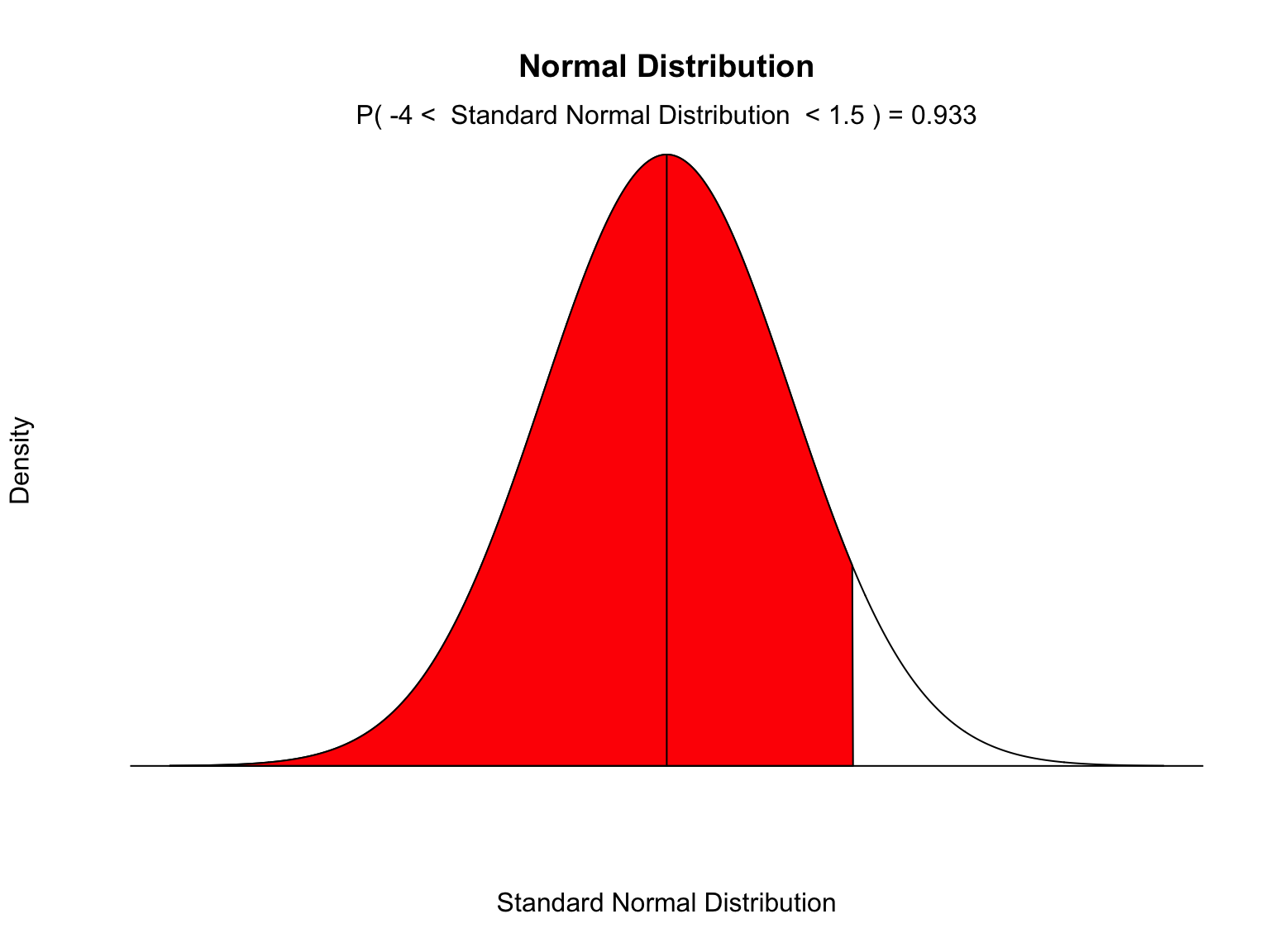# Functions

2/6/2019

library(tidyverse)
## ── Attaching packages ──── tidyverse 1.2.1 ──
## ✔ ggplot2 3.0.0     ✔ purrr   0.2.5
## ✔ tibble  1.4.2     ✔ dplyr   0.7.6
## ✔ tidyr   0.8.1     ✔ stringr 1.3.1
## ✔ readr   1.1.1     ✔ forcats 0.3.0
## ── Conflicts ─────── tidyverse_conflicts() ──
## ✖ dplyr::lag()    masks stats::lag()

## Range

The range function in R does not do exactly what we want. The range of a numeric variable in statistics is the difference between the maximum and the minimum value of the variable. But the range function in R returns the minimu and maximum values, but doesn’t do the subtraction. I’ll create a function myRange, which will do the subtraction.

myRange = function(x){
# x needs to be a numeric vector.
# Remove any NA values.
x = x[!is.na(x)]
return(max(x) - min(x))
}

Now compare the results of this function and the base R function range().

range(mtcars$mpg) ##  10.4 33.9 myRange(mtcars$mpg)
##  23.5

## Fixing summary()

The standard function summary leaves out some standard descriptivs statistics of a numeric variable. We can create our own function mySummary to do what we want.

mySummary = function(x){
# x needs to be a numeric vector.
# Remove any NA values.
x = x[!is.na(x)]

result = summary(x)
result = c(result,sd(x))
result = c(result,myRange(x))
result = c(result,IQR(x))
names(result) = "sd"
names(result) = "range"
names(result) = "IQR"

return(result)
}
mySummary(mtcars$mpg) ## Min. 1st Qu. Median Mean 3rd Qu. Max. sd ## 10.400000 15.425000 19.200000 20.090625 22.800000 33.900000 6.026948 ## range IQR ## 23.500000 7.375000 ## myNormProb This is a function I wrote to display the results of normal probability calculations. It’s a good example of the usage of arguments. MyNormProb <- function( lb = NA, # Lower bound ub = NA, # Upper bound mean = 0, # Mean sd = 1, # Standard deviation MyLabel = "Standard Normal RV" # Description of the variable # This function produces a plot and the probability that # a normal random variable with the given mean and # standrard deviation falls between the given lower and # upper bounds. ) { if (is.na(lb)) {lb <- mean - 4 * sd} if (is.na(ub)) {ub <- mean + 4 * sd} x <- seq(-4,4,length=1000)*sd + mean hx <- dnorm(x,mean,sd) plot(x, hx, type="n", xlab=MyLabel, ylab="Density", main="Normal Distribution", axes=FALSE) i <- x >= lb & x <= ub lines(x, hx) polygon(c(lb,x[i],ub), c(0,hx[i],0), col="red") area <- pnorm(ub, mean, sd) - pnorm(lb, mean, sd) result <- paste("P(",lb,"< ",MyLabel," <" ,ub,") =", signif(area, digits=3)) mtext(result,3) abline(0,0) segments(x0 = mean, y0 = 0, x1 = mean, y1 = dnorm(mean,mean,sd)) return(area) } v <- MyNormProb(ub=1.5,MyLabel="Standard Normal Distribution")## Exercise The functions IQR and myRange are similar. What do they have in common? Can we parameterize the common feature and use it to build a generalized function? midXX = function(x,XX){ # x is a numeric vector # XX is the middle percentage # the function returns the length # of the interval covered by the middle # XX% of the data in x. dec = XX/100 lb = .5*(1-dec) ub = dec + lb midrange = (quantile(x,ub) - quantile(x,lb)) names(midrange) = "" return(midrange) } nrv = rnorm(1000) midXX(nrv,50) ## ## 1.392344 IQR(nrv) ##  1.392344 ## Exercise Let’s load the olywthr data and look at the first 15 days of February. ## Solution load("~/Downloads/olywthr.rdata") earlyFeb = filter(olywthr,mo == 2 & dy >= 1 & dy <= 15) ## Exercise What is the probability that snow occurs on a date in this range? ## Solution mean(earlyFeb$SNOW>0)
##  0.04529617

## Exercise

What is the probability that the minimum temperature on a date in this range is below 32 degrees?

## Solution

mean(earlyFeb\$TMIN < 32)
##  0.4156297